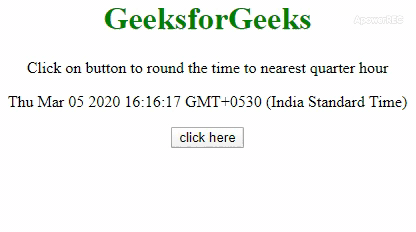GeeksforGeeks App
Open AppBrowser
Continue

# How to Round Time to the Nearest Quarter Hour using JavaScript ?

We have given a time and the task is to round the time to the nearest quarter-hour with the help of JavaScript. There are two approaches that are discussed below:

Approach 1: Use getMinutes() and getHours() methods to get the minutes and hours in a variable (Ex. mins, hrs) add the 7.5 to mins, divide it by 15, take the integer value and multiply it by 15, which will give the nearest quarter of minutes. If this value goes beyond 60, then take the mod with 60. For hrs, if mins 52 and if the hrs == 23, set hrs to 0 else increment its value by 1.

• Example:

 ```<``html``>`` ` `<``head``>``    ``<``title``>``        ``Round time to the nearest quarter ``        ``hour in JavaScript``    ````    ``<``style``>``        ``body {``            ``text-align: center;``        ``}``         ` `        ``h1 {``            ``color: green;``        ``}``    `````` ` `<``body``>``    ``<``h1``> ``       ``GeeksforGeeks ``    ````    ``<``p``>``      ``Click on button to round the time ``      ``to nearest quarter hour``    ````    ``<``p` `id``=``"gfg"``>``    ``<``button` `onClick``=``"GFG_Fun()"``>``      ``click here``    ````    ``<``p` `id``=``"geeks"``>``    ``<``script``>``        ``var up = document.getElementById('gfg');``        ``var down = document.getElementById('geeks');``        ``var date = new Date();``        ``up.innerHTML = "" + date + "";`` ` `        ``function GFG_Fun() {`` ` `            ``// Getting minutes``            ``var mins = date.getMinutes();`` ` `            ``// Getting hours``            ``var hrs = date.getHours();``            ``var m = (parseInt((mins + 7.5) / 15) * 15) % 60;`` ` `            ``// Converting '09:0' to '09:00'``            ``m = m < ``10` `? '0' + m : m;``            ``var ``h` `= ``mins` `> 52 ? (hrs === 23 ? 0 : ++hrs) : hrs;`` ` `            ``// Converting '9:00' to '09:00'``            ``h = h < ``10` `? '0' + h : h;``            ``down.innerHTML` `= ``h` `+ ":" + m;``        ``}``    `````` ` ``

• Output:Approach 2: Use getMinutes() and getHours() methods to get the minutes and hours in a variable (Ex. mins, hrs). Divide mins by 15, take the round value by Math.round() and multiply it by 15, which will give the nearest quarter of minutes. If this value goes beyond 60, then take the mod with 60. For hrs, if mins 52 and if the hrs == 23, set hrs to 0 else increment its value by 1.

• Example:

 ```<``html``>`` ` `<``head``>``    ``<``title``>``        ``Round time to the nearest quarter ``        ``hour in JavaScript``    ````    ``<``style``>``        ``body {``            ``text-align: center;``        ``}``         ` `        ``h1 {``            ``color: green;``        ``}``    `````` ` `<``body``>``    ``<``h1``> ``       ``GeeksforGeeks ``    ````    ``<``p``>``      ``Click on button to round the time ``      ``to nearest quarter hour``    ````    ``<``p` `id``=``"gfg"``>``    ``<``button` `onClick``=``"GFG_Fun()"``>``      ``click here``    ````    ``<``p` `id``=``"geeks"``>``    ``<``script``>``        ``var up = document.getElementById('gfg');``        ``var down = document.getElementById('geeks');``        ``var date = new Date();``        ``up.innerHTML = "" + date + "";`` ` `        ``function GFG_Fun() {`` ` `            ``// Getting minutes``            ``var mins = date.getMinutes();`` ` `            ``// Getting hours``            ``var hrs = date.getHours();``            ``var m = (Math.round(mins/15) * 15) % 60;`` ` `            ``// Converting '09:0' to '09:00'``            ``m = m < ``10` `? '0' + m : m;``            ``var ``h` `= ``mins` `> 52 ? (hrs === 23 ? 0 : ++hrs) : hrs;`` ` `            ``// Converting '9:00' to '09:00'``            ``h = h < ``10` `? '0' + h : h;``            ``down.innerHTML` `= ``h` `+ ":" + m;``        ``}``    `````` ` ``

• Output:My Personal Notes arrow_drop_up
Related Tutorials